• 将2D高斯核函数根据非正交方向进行分解，得到一维的x轴和其他方向的高斯函数，通过统计学方法，将分解的高斯函数与卷积滤波器或者迭代滤波器结合进行边缘检测。
• self.KernalMatrix = self.CalKernalMatrix() # 高斯核矩阵 self.alpha = np.zeros(self.m) # 初始化拉格朗日向量，长度为训练集样本容量 self.b = 0 # 初始化参数b self.C = C # 惩罚参数 self.toler = toler # ...

'''支持向量机-------------------------------------数据集：Mnist训练集数量：10000测试集数量：1000---------------------------------训练结果：初始化时间：327s训练时间：285s正确率：0.99'''

import numpy as np

import time

from itertools import islice

import math

# 打开数据文件

fr = open(file, 'r')

# 准备两个list存储data和label

data = []

label = []

# 逐行读取数据，使用islice可以直接跳过第一行表头进行逐行读取

for line in islice(fr,1,None):

# 对每一行消去空格，并根据','进行分割

splited = line.strip().split(',')

# 分割后的第一个元素是Label，跳过label遍历所有特征值

# 归一化

int_line = [int(num) / 255 for num in splited[1:]]

# 逐行存储数据

data.append(int_line)

# 将问题转换为二分类问题

if int(splited) == 0:

label.append(1)

else:

label.append(-1)

# 转换成ndarray形式方便后续计算

data = np.array(data)

label = np.array(label)

# 返回数据的特征部分和标记部分

return data, label

'''定义SVM类'''

class SVM:

'''初始化参数'''

def __init__(self, train_data, train_label, sigma, C, toler, itertime):

self.train_data = train_data # 训练集数据

self.train_label = train_label # 训练集标记

self.m, self.n = np.shape(train_data) # self.m为训练集样本容量，self.n为特征数量

self.sigma = sigma # 高斯核分母上的超参数

self.KernalMatrix = self.CalKernalMatrix() # 高斯核矩阵

self.alpha = np.zeros(self.m) # 初始化拉格朗日向量，长度为训练集样本容量

self.b = 0 # 初始化参数b

self.C = C # 惩罚参数

self.toler = toler # 松弛变量

self.itertime = itertime # 迭代次数

self.E = [float(-1 * y) for y in self.train_label] # 初始化Elist，因为alpha和b初始值为0，因此E的初始值为训练集标记的值

'''计算高斯核矩阵'''

def CalKernalMatrix(self):

# 初始化高斯核矩阵，矩阵为m*m大小

KernalMatrix = [[0 for i in range(self.m)] for j in range(self.m)]

# 遍历每一个样本

for i in range(self.m):

# 首先得到一个样本的数据

X = self.train_data[i]

# 仅遍历从i到self.m的样本，因为Kij和Kji的值相同，所以只需要计算一次

for j in range(i, self.m):

# 得到另一个样本的数据

Z = self.train_data[j]

# 计算核函数

K = np.exp(-1 * np.dot(X - Z, X - Z) / (2 * np.square(self.sigma)))

# 存储在核矩阵中对称的位置

KernalMatrix[i][j] = K

KernalMatrix[j][i] = K

KernalMatrix = np.array(KernalMatrix)

return KernalMatrix

'''计算g(xi)'''

def Calgxi(self, i):

Index = [index for index, value in enumerate(self.alpha) if value != 0]

gxi = 0

for index in Index:

gxi += self.alpha[index] * self.train_label[index] * self.KernalMatrix[i][index]

gxi = gxi + self.b

return gxi

'''判断是否符合KKT条件'''

def isSatisfyKKT(self, i):

# 获得alpha[i]的值

alpha_i = self.alpha[i]

# 计算yi * g(xi)

gxi = self.Calgxi(i)

yi = self.train_label[i]

yi_gxi = yi * gxi

# 判断是否符合KKT条件

if -1 * self.toler < alpha_i < self.toler and yi_gxi >= 1:

return True

elif -1 * self.toler < alpha_i < self.C + self.toler and math.fabs(yi_gxi - 1) < self.toler:

return True

elif self.C - self.toler < alpha_i < self.C + self.toler and yi_gxi <= 1:

return True

return False

'''SMO算法'''

def SMO(self):

# 迭代

t = 0

parameterchanged = 1

while t < self.itertime and parameterchanged > 0:

t += 1

parameterchanged = 0

'''选择两个alpha'''

# 外层循环，选择第一个alpha

for i in range(self.m):

# 判断是否符合KKT条件，如果不满足，则选择该alpha为alpha1

# 如果满足，则继续外层循环

TorF = self.isSatisfyKKT(i)

if TorF == False:

alpha1 = self.alpha[i]

# 从Earray得到alpha1对应的E1

E1 = self.E[i]

# 复制一个EMatrix，并令E1的位置为nan

# 这样在接下来找最大值和最小值时将不会考虑E1

# 这里需要使用copy，如果不用copy，改变EM_temp也会同时改变EMatrix

EM_temp = np.copy(self.E)

EM_temp[i] = np.nan

# 我们需要使|E1-E2|的值最大，由此选择E2

# 首先初始化maxE1_E2和E2及E2的下标j

maxE1_E2 = -1

E2 = np.nan

j = -1

# 内层循环

# 遍历EM_temp中的每一个Ei，得到使|E1-E2|最大的E和它的下标

for j_temp, Ej in enumerate(EM_temp):

if math.fabs(E1 - Ej) > maxE1_E2:

maxE1_E2 = math.fabs(E1 - Ej)

E2 = Ej

j = j_temp

# alpha2为E2对应的alpha

alpha2 = self.alpha[j]

'''求最优alpha1和alpha2'''

y1 = self.train_label[i]

y2 = self.train_label[j]

# 计算η

K11 = self.KernalMatrix[i][i]

K22 = self.KernalMatrix[j][j]

K12 = self.KernalMatrix[i][j]

eta = K11 + K22 - 2 * K12

# 计算alpha2_new

alpha2_new = alpha2 + y2 * (E1 - E2) / eta

# 计算上限H和下限L

if y1 != y2:

L = max([0, alpha2 - alpha1])

H = min([self.C, self.C + alpha2 - alpha1])

else:

L = max([0, alpha2 + alpha1 - self.C])

H = min([self.C, alpha2 + alpha1])

# 剪切alpha2_new

if alpha2_new > H:

alpha2_new = H

elif alpha2_new < L:

alpha2_new = L

# 得到alpha1_new

alpha1_new = alpha1 + y1 * y2 * (alpha2 - alpha2_new)

'''更新b'''

# 计算b1_new和b2_new

b1_new = -1 * E1 - y1 * K11 * (alpha1_new - alpha1) \

- y2 * K12 * (alpha2_new - alpha2) + self.b

b2_new = -1 * E2 - y1 * K12 * (alpha1_new - alpha1) \

- y2 * K22 * (alpha2_new - alpha2) + self.b

# 根据alpha1和alpha2的范围确定b_new

if 0 < alpha1_new < self.C and 0 < alpha2_new < self.C:

b_new = b1_new

else:

b_new = (b1_new + b2_new) / 2

'''更新E'''

# 首先需要更新两个alpha和b

self.alpha[i] = alpha1_new

self.alpha[j] = alpha2_new

self.b = b_new

# 计算Ei_new和Ej_new

E1_new = self.Calgxi(i) - y1

E2_new = self.Calgxi(j) - y2

# 更新E

self.E[i] = E1_new

self.E[j] = E2_new

if math.fabs(alpha2_new - alpha2) >= 0.000001:

parameterchanged += 1

print('itertime: %a parameterchanged: %a' % (t,parameterchanged))

# 最后遍历一遍alpha，大于0的下标即对应支持向量

VecIndex = [index for index, value in enumerate(self.alpha) if value > 0]

# 返回支持向量的下标，之后在预测时还需要用到

return VecIndex

'''计算b'''

def OptimizeB(self):

for j, a in enumerate(self.alpha):

if 0 < a < self.C:

break

yj = self.train_label[j]

summary = 0

for i in range(self.alpha):

summary += self.alpha[i] * self.train_label[i] * self.KernalMatrix[i][j]

optimiezedB = yj - summary

self.b = optimiezedB

'''计算单个核函数'''

def CalSingleKernal(self, x, z):

SingleKernal = np.exp(-1 * np.dot(x - z, x - z) / (2 * np.square(self.sigma)))

return SingleKernal

'''单个新输入实例的预测'''

def predict(self, x, VecIndex):

# 决策函数计算

# 求和项初始化

summary = 0

# Index中存储着不为0的alpha的下标

for i in VecIndex:

alphai = self.alpha[i]

yi = self.train_label[i]

Kernali = self.CalSingleKernal(x, self.train_data[i])

summary += alphai * yi * Kernali

# 最后+b

# np.sign得到符号

result = np.sign(summary + self.b)

return result

'''测试模型'''

def test(self, test_data, test_label, VecIndex):

# 测试集实例数量

TestNum = len(test_label)

errorCnt = 0

# 对每一个实例进行预测

for i in range(TestNum):

result = self.predict(test_data[i], VecIndex)

if result != test_label[i]:

errorCnt += 1

Acc = 1 - errorCnt / TestNum

return Acc

'''测试模型'''

if __name__ == "__main__":

# 输入训练集

# 输入测试集

# 初始化

print('start initiating')

start = time.time()

svm = SVM(train_data[0:10000], train_label[0:10000], 10, 200, 0.001, 10)

end = time.time()

print('end initiating')

print('initiating time: ', end - start)

# 开始SMO算法

print('start training')

start = time.time()

VecIndex = svm.SMO()

end = time.time()

print('end training')

print('training time: ', end - start)

# 开始测试模型

Acc = svm.test(test_data[0:1000], test_label[0:1000], VecIndex)

print('Accurate: ', Acc)

展开全文• 一、核函数（Kernel Function）1）格式K(x, y)：表示样本 x 和 y，添加多项式特征得到新的样本 x'、y'，K(x, y) 就是返回新的样本经过计算得到的值；在 SVM 类型的算法 SVC() 中，K(x, y) 返回点乘：x' . y' 得到的...

一、核函数（Kernel Function）

1）格式

K(x, y)：表示样本 x 和 y，添加多项式特征得到新的样本 x'、y'，K(x, y) 就是返回新的样本经过计算得到的值；

在 SVM 类型的算法 SVC() 中，K(x, y) 返回点乘：x' . y' 得到的值；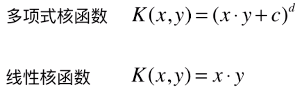2）多项式核函数

业务问题：怎么分类非线性可分的样本的分类？

内部实现：

对传入的样本数据点添加多项式项；

新的样本数据点进行点乘，返回点乘结果；

多项式特征的基本原理：依靠升维使得原本线性不可分的数据线性可分；

升维的意义：使得原本线性不可分的数据线性可分；

例：

一维特征的样本，两种类型，分布如图，线性不可分：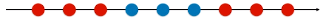为样本添加一个特征：x2 ，使得样本在二维平面内分布，此时样本在 x 轴升的分布位置不变；如图，可以线性可分：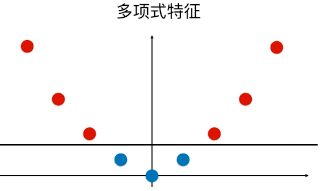3）优点 / 特点

不需要每次都具体计算出原始样本点映射的新的无穷维度的样本点，直接使用映射后的新的样本点的点乘计算公式即可；

减少计算量

减少存储空间

一般将原始样本变形，通常是将低维的样本数据变为高维数据，存储高维数据花费较多的存储空间；使用核函数，不用考虑原来样本改变后的样子，也不用存储变化后的结果，只需要直接使用变化的结果进行运算并返回运算结果即可；

核函数的方法和思路不是 SVM 算法特有，只要可以减少计算量和存储空间，都可以设计核函数方便运算；

对于比较传统的常用的机器学习算法，核函数这种技巧更多的在 SVM 算法中使用；

4）SVM 中的核函数

svm 类中的 SVC() 算法中包含两种核函数：

SVC(kernel = 'ploy')：表示算法使用多项式核函数；

SVC(kernel = 'rbf')：表示算法使用高斯核函数；

SVM 算法的本质就是求解目标函数的最优化问题；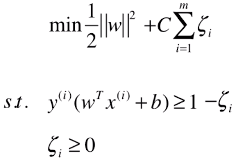求解最优化问题时，将数学模型变形：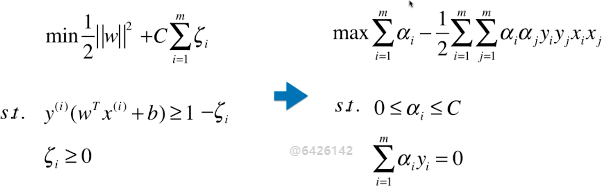5）多项式核函数

格式：

from sklearn.svm importSVC

svc= SVC(kernel = 'ploy')

思路：设计一个函数（ K(xi, xj) ），传入原始样本（x(i) 、 x(j)），返回添加了多项式特征后的新样本的计算结果（x'(i) . x'(j)）；

内部过程：先对 xi 、xj 添加多项式，得到：x'(i) 、 x'(j)，再进行运算：x'(i) . x'(j) ；

x(i) 添加多项式特征后：x'(i) ；

x(j) 添加多项式特征后：x'(j) ；

x(i) .x(j)转化为：x'(i) .x'(j) ；

其实不使用核函数也能达到同样的目的，这里核函数相当于一个技巧，更方便运算；

二、高斯核函数（RBF）

业务问题：怎么分类非线性可分的样本的分类？

1）思想

业务的目的是样本分类，采用的方法：按一定规律统一改变样本的特征数据得到新的样本，新的样本按新的特征数据能更好的分类，由于新的样本的特征数据与原始样本的特征数据呈一定规律的对应关系，因此根据新的样本的分布及分类情况，得出原始样本的分类情况。

应该是试验反馈，将样本的特征数据按一定规律统一改变后，同类样本更好的凝聚在了一起；

高斯核和多项式核干的事情截然不同的，如果对于样本数量少，特征多的数据集，高斯核相当于对样本降维；

高斯核的任务：找到更有利分类任务的新的空间。

方法：类似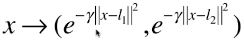的映射。

高斯核本质是在衡量样本和样本之间的“相似度”，在一个刻画“相似度”的空间中，让同类样本更好的聚在一起，进而线性可分。

疑问：

“衡量”的手段，经过这种映射之后，为什么同类样本能更好的分布在一起？

2）定义方式x、y：样本或向量；

γ：超参数；高斯核函数唯一的超参数；

|| x - y ||：表示向量的范数，可以理解为向量的模；

表示两个向量之间的关系，结果为一个具体值；

高斯核函数的定义公式就是进行点乘的计算公式；

3）功能

先将原始的数据点（x， y）映射为新的样本（x'，y'）；

再将新的特征向量点乘（x' . y'），返回其点乘结果；

计算点积的原因：此处只针对 SVM 中的应用，在其它算法中的应用不一定需要计算点积；4）特点

高斯核运行开销耗时较大，训练时间较长；

一般使用场景：数据集 (m, n)，m < n；

一般应用领域：自然语言处理；

自然语言处理：通常会构建非常高维的特征空间，但有时候样本数量并不多；

5）高斯函数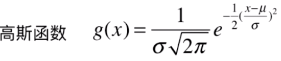正态分布就是一个高斯函数；

高斯函数和高斯核函数，形式类似；

6）其它

高斯核函数，也称为 RBF 核（Radial Basis Function Kernel），也称为径向基函数；

高斯核函数的本质：将每一个样本点映射到一个无穷维的特征空间；

无穷维：将 m*n 的数据集，映射为 m*m 的数据集，m 表示样本个数，n 表示原始样本特征种类，样本个数是无穷的，因此，得到的新的数据集的样本也是无穷维的；

高斯核升维的本质，使得线性不可分的数据线性可分；

三、RBF 转化特征数据原理

1）转化原理x：需要改变维度的样本；

np.array([l1, l2, ..., lm])== X == np.array([x1, x2, ... , xm])：Landmark，地标，一般直接选取数据集 X 的所有样本作为地标；（共 m 个）

对于 (m, n) 的数据集：转化为 (m, m) 的数据集；将 n 维的样本转化为 m 维的样本；

对于原始数据集中的每一个样本 x，也可以有几个地标点，就将 x 转化为几维；

2）主要为两部分

先将原始的数据点映射为一种新的特征向量，再将新的特征向量点乘，返回其点乘结果；

维度转化：样本 x1 转化x1' ：(e-γ||x1 - x1||**2, e-γ||x1 - x2||**2, e-γ||x1 - x3||**2, ..., e-γ||x1 - xm||**2)，同理样本x2 的转化 x2'；（地标点就是数据集 X 的样本点）

点乘计算：x1' . x2' == K(x1, x2) == e-γ||x1 - x2||**2，最终结果为一个具体值；

3）实例模拟维度转化过程

一维升到二维

原始样本分布：第一步：选取地标点：L1、L2 ；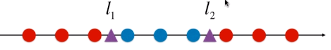第二步：升维计算四、程序模拟

目的：将线性不可分的数据变为线性可分；

方法：一维数据升到二维；

1）模拟数据集

x 数据集：每一个样本只有一个特征，且分布规律线性不可分；

np.arange(m, n, l)：将区间 [m, n) 按间距为 l 等分，等分后的数据点包含 m 值，不包含 n；

*len(x[y==0])： 是一个 list，list * C 表示将列表复制 C 份；

如：*5 == [0, 0, 0, 0, 0]

importnumpy as npimportmatplotlib.pyplot as plt

x= np.arange(-4, 5, 1)

y= np.array((x >= -2) & (x <= 2), dtype='int')

plt.scatter(x[y==0], *len(x[y==0]))

plt.scatter(x[y==1], *len(x[y==1]))

plt.show()2）经过高斯核，得到新的数据集

np.exp(m)：表示 e 的 m 次幂；

np.empty(元组)：（元组）=(m, n)，生成一个 m 行 n 列的空的矩阵；

enumerate(iterator)：返回可迭代对象的 index 和 value；

for i, data in enumerate(x)：i 存放向量 x 的 index，data 存放向量 x 的 index 对应的元素值；

defgaussian(x, l):#此处直接将超参数 γ 设定为 1.0；

#此处 x 表示一维的样本，也就是一个具体的值，l 相应的也是一个具体的数，因为 l 和 x 一样，从特征空间中选定；

gamma = 1.0

#此处因为 x 和 l 都只是一个数，不需要再计算模，可以直接平方；

return np.exp(-gamma * (x-l)**2)#设定地标 l1、l2 为 -1和1

l1, l2 = -1, 1x_new= np.empty((len(x), 2))for i, data inenumerate(x):

x_new[i, 0]=gaussian(data, l1)

x_new[i,1] =gaussian(data, l2)

plt.scatter(x_new[y==0, 0], x_new[y==0, 1])

plt.scatter(x_new[y==1, 0], x_new[y==1, 1])

plt.show()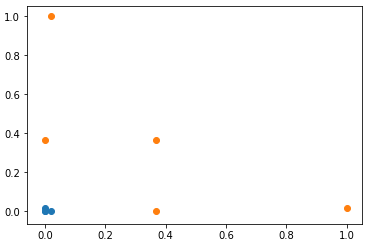展开全文• 二维高斯核函数(python)

万次阅读 2017-12-12 18:38:13
二维高斯核函数python

今天突然想要去试一试opencv里面的高斯模糊怎么实现，虽然以前已经写过很多次关于高斯核的函数了，但是有个好奇点，那就是一般不填sigma这个参数的时候，opencv是怎么计算的。

关于具体的高斯函数的讲解，已经有人写的很详细了，我就不赘述了，所以给大家个链接有兴趣的可以去看看。

http://www.ruanyifeng.com/blog/2012/11/gaussian_blur.html

我这里想做的实验是到底opencv中的高斯模糊函数GaussianBlur使用的高斯核是什么。

但是直接获取这个二维的高斯核貌似是不可行的，opencv提供了一维高斯核获取的函数。

具体参考以下页面

https://docs.opencv.org/2.4.13.2/modules/imgproc/doc/filtering.html#void sepFilter2D(InputArray src, OutputArray dst, int ddepth, InputArray kernelX, InputArray kernelY, Point anchor, double delta, int borderType)

那么此处问题就出来了，如何通过一维高斯核生成二维高斯核。

先获取两个一维高斯核，而后对后一个高斯核进行转置，而后第一个高斯核核第二个高斯核通过矩阵相乘就可以得到一个二维高斯核了。

下面是测试代码

def gaussian_kernel_2d_opencv(kernel_size = 3,sigma = 0):
kx = cv2.getGaussianKernel(kernel_size,sigma)
ky = cv2.getGaussianKernel(kernel_size,sigma)
return np.multiply(kx,np.transpose(ky))

然后我需要的是自己写一个二维高斯核函数，此处写法有很多版本，但是我是参考了刚才上面给出的参考链接中的计算方式，同时参考了opencv的源码。

两个地方不一样，一个是opencv当中在计算高斯模版的时候并没有在最后计算除以pi2(sigma**2)，而是将每次计算后的数值加起来，最后用1/总和，然后输出的时候将刚才计算的数值乘上本身的像素值。

本来此处写一下公式会比较好理解，但是这里稍微偷懒一下就不给出具体的公式了，大家自行看我下面的代码就OK了。

#kernel_size set (n,n) default
def gaussian_2d_kernel(kernel_size = 3,sigma = 0):

kernel = np.zeros([kernel_size,kernel_size])
center = kernel_size//2

if sigma == 0:
sigma = ((kernel_size-1)*0.5 - 1)*0.3 + 0.8

s = 2*(sigma**2)
sum_val = 0
for i in range(0,kernel_size):
for j in range(0,kernel_size):
x = i-center
y = j-center
kernel[i,j] = np.exp(-(x**2+y**2) / s)
sum_val += kernel[i,j]
#/(np.pi * s)
sum_val = 1/sum_val
return kernel*sum_val

这个计算结果和通过opencv获取一维高斯核通过计算转换为二维高斯核的结果完全一致。此处实验成功。

但是！！通过这个高斯核和图像进行卷积操作的结果和直接通过opencv调用GaussianBlur计算的结果是有所不同的。虽然单纯从数值上来讲，基本都只是差1的大小，但是这说明此函数并不是直接和这个卷积核进行卷积的，而是还做了一点修改。那么具体是什么，我暂时没画时间去查源码，如有了解者希望能够告知我，感激不尽。

最后一个小注意点，上面忘记书写了，卷积核大小在小于等于7的情况下结果是和我自己写的那个函数计算不完全一致的。原因在于opencv源码当中小于等于7的高斯核并不是通过计算给出而是直接给出了结果，所以在小数上有一定差距，估计是效率问题才这么做的。

20190115 修正（源自评论“临京雪月”）

opencv源码当中小于等于7的高斯核并不是通过计算给出而是直接给出了结果。这句话不对，当小于等于7且sigma<=0时候才会使用内置的模板，其余情况同样是计算出来的结果

展开全文python opencv 高斯模糊
• import numpy as np from scipy.spatial.distance import cdist from scipy.optimize import minimize RBF kernel k(x,y)=exp(−∣∣x−y∣∣22σ2) k(x,y) = exp(-\frac{||x - y||^2}{2\sigma^2}) ...
import numpy as np
from scipy.spatial.distance import cdist
from scipy.optimize import minimize

RBF kernel

k ( x , y ) = e x p ( − ∣ ∣ x − y ∣ ∣ 2 2 σ 2 ) k(x,y) = exp(-\frac{||x - y||^2}{2\sigma^2})

The function rbf_kernel computes the radial basis function (RBF) kernel between two vectors. This kernel is defined as:
k ( x , y ) = e x p ( − γ ∣ ∣ x − y ∣ ∣ 2 ) k(x,y) = exp(-\gamma||x - y||^2)
where x x and y y are the input vectors. If γ = σ − 2 \gamma = \sigma^{-2} the kernel is known as the Gaussian kernel of variance σ − 2 \sigma^{-2} .

The euclidean distance between a pair of rowvector x and y is computed as:
d i s t ( x , y ) = s q r t ( d o t ( x , x ) − 2 ∗ d o t ( x , y ) + d o t ( y , y ) ) dist(x,y)= sqrt(dot(x,x)-2 * dot(x,y)+ dot(y,y))
This formulation has two advantages over other ways of computing distances.

1. First, it is computationally efficient when dealing with sparse data.
2. Second, if one argument varies but the other remains unchanged, then dot(x, x) and/or dot(y, y) can be pre-computed.

There are four ways to obtain kernel matrixs.

• According to the theory.
def rbf_kernel_1(X,Y,gamma = 0.1):
"""
param X: ndarray of shape (n_samples_X,n_features)
param Y: ndarray of shape (n_samples_Y,n_features)
param gamma: if None, default to 0.1, refer to 1 / n_features
Return: kernel_matrix: ndarray of shape(n_samples_X,n_samples_Y)
"""
#compute X_norm_squared,Y_norm_squared
if X.ndim == 1: #dim of X, if dim_x = 1
X_norm_squared = X **2
Y_norm_squared = Y **2
else: #dim >= 2
X_norm_squared = (X **2).sum(axis = 1).reshape(X.shape,1)
Y_norm_squared = (Y **2).sum(axis = 1).reshape(Y.shape,1)
#X_norm_squared : array-like of shape (n_samples_X,)
#Y_norm_squared : array-like of shape (n_samples_Y,)
squared_Euclidean_distances = Y_norm_squared[:,] + X_norm_squared.T - 2 * np.dot(Y,X.T)
return np.exp(-squared_Euclidean_distances * gamma)

• by using scipy distance cdist to get Euclidean distances
def rbf_kernel_2(X,Y,gamma = 0.1):
if X.ndim==X.ndim and X.ndim==2: # both matrices
return np.exp(-gamma * cdist(X,Y)**2)
else: # both vectors or a vector and a matrix
return np.exp(- gamma * ( dot(X,X.T) + dot(Y,Y.T)- 2*dot(X,Y)))

• The most clear and rough way.
def rbf_kernel_3(X,Y, gamma = 0.1):
dist_matrix = np.sum(X**2, 1).reshape(-1, 1) + np.sum(Y**2, 1) - 2 * np.dot(X,Y.T)
return np.exp(-gamma * dist_matrix)

• rbf_kernel reference from sklearn
from sklearn.metrics.pairwise import rbf_kernel
def rbf_kernel_4(X,Y,gamma = 0.1):
return rbf_kernel(X,Y,gamma)

Having a test as follows.

if "__name__" == "main":
n = 100
dim = 1
X = np.array(np.linspace(1,10,n)).reshape(n,dim)
Y = np.array(np.linspace(1,10,n)).reshape(n,dim)
print(rbf_kernel_1(X,Y,gamma = 0.1))
print("----------------------------------------------------")
print(rbf_kernel_2(X,Y,gamma = 0.1))
print("----------------------------------------------------")
print(rbf_kernel_3(X,Y,gamma = 0.1))
print("----------------------------------------------------")
print(rbf_kernel_4(X,Y,gamma = 0.1))

[[1.00000000e+00 9.99173895e-01 9.96699673e-01 ... 4.19673698e-04
3.57208797e-04 3.03539138e-04]
[9.99173895e-01 1.00000000e+00 9.99173895e-01 ... 4.92247497e-04
4.19673698e-04 3.57208797e-04]
[9.96699673e-01 9.99173895e-01 1.00000000e+00 ... 5.76417873e-04
4.92247497e-04 4.19673698e-04]
...
[4.19673698e-04 4.92247497e-04 5.76417873e-04 ... 1.00000000e+00
9.99173895e-01 9.96699673e-01]
[3.57208797e-04 4.19673698e-04 4.92247497e-04 ... 9.99173895e-01
1.00000000e+00 9.99173895e-01]
[3.03539138e-04 3.57208797e-04 4.19673698e-04 ... 9.96699673e-01
9.99173895e-01 1.00000000e+00]]
----------------------------------------------------
[[1.00000000e+00 9.99173895e-01 9.96699673e-01 ... 4.19673698e-04
3.57208797e-04 3.03539138e-04]
[9.99173895e-01 1.00000000e+00 9.99173895e-01 ... 4.92247497e-04
4.19673698e-04 3.57208797e-04]
[9.96699673e-01 9.99173895e-01 1.00000000e+00 ... 5.76417873e-04
4.92247497e-04 4.19673698e-04]
...
[4.19673698e-04 4.92247497e-04 5.76417873e-04 ... 1.00000000e+00
9.99173895e-01 9.96699673e-01]
[3.57208797e-04 4.19673698e-04 4.92247497e-04 ... 9.99173895e-01
1.00000000e+00 9.99173895e-01]
[3.03539138e-04 3.57208797e-04 4.19673698e-04 ... 9.96699673e-01
9.99173895e-01 1.00000000e+00]]
----------------------------------------------------
[[1.00000000e+00 9.99173895e-01 9.96699673e-01 ... 4.19673698e-04
3.57208797e-04 3.03539138e-04]
[9.99173895e-01 1.00000000e+00 9.99173895e-01 ... 4.92247497e-04
4.19673698e-04 3.57208797e-04]
[9.96699673e-01 9.99173895e-01 1.00000000e+00 ... 5.76417873e-04
4.92247497e-04 4.19673698e-04]
...
[4.19673698e-04 4.92247497e-04 5.76417873e-04 ... 1.00000000e+00
9.99173895e-01 9.96699673e-01]
[3.57208797e-04 4.19673698e-04 4.92247497e-04 ... 9.99173895e-01
1.00000000e+00 9.99173895e-01]
[3.03539138e-04 3.57208797e-04 4.19673698e-04 ... 9.96699673e-01
9.99173895e-01 1.00000000e+00]]
----------------------------------------------------
[[1.00000000e+00 9.99173895e-01 9.96699673e-01 ... 4.19673698e-04
3.57208797e-04 3.03539138e-04]
[9.99173895e-01 1.00000000e+00 9.99173895e-01 ... 4.92247497e-04
4.19673698e-04 3.57208797e-04]
[9.96699673e-01 9.99173895e-01 1.00000000e+00 ... 5.76417873e-04
4.92247497e-04 4.19673698e-04]
...
[4.19673698e-04 4.92247497e-04 5.76417873e-04 ... 1.00000000e+00
9.99173895e-01 9.96699673e-01]
[3.57208797e-04 4.19673698e-04 4.92247497e-04 ... 9.99173895e-01
1.00000000e+00 9.99173895e-01]
[3.03539138e-04 3.57208797e-04 4.19673698e-04 ... 9.96699673e-01
9.99173895e-01 1.00000000e+00]]

展开全文• 径向基/RBF/高斯核 都是这种形式 为了感性理解，模拟一下。。。过程 高斯核本质 l1 l2 两个地标 ...本质是将原本数据，映射到一个无穷维空间（样本理论上有无穷多个） 计算开销特别大~~...#直观理解高斯核函数 import .
• 机器学习算法Python实现目录1、代价函数其中：下面就是要求出theta，使代价最小，即代表我们拟合出来的方程距离真实值最近共有m条数据，其中代表我们要拟合出来的方程到真实值距离的平方，平方的原因是因为可能有...
• 二次项K函数的计算方法 d代表degree，用多项式核函数的方法计算新的矩阵 RBFKernel（高斯核函数） gamma为高斯核的超参数def RBFKernelSVC(gamma=1.0): return Pipeline([ ("std_sacler",StandardScaler()), ("svc...
• Python实现机器学习算法

千次阅读 2020-12-02 14:23:47
编辑推荐:本文来自于CSDN，介绍了机器学习算法Python实现的逻辑回归、BP神经网络、K-Means聚类算法以及多元高斯分布等相关知识。一、线性回归1、代价函数其中：下面就是要求出theta，使代价最小，即代表我们拟合出来...
• 单类SVM：SVDD

2020-12-02 14:23:48
高斯核 高斯核函数的表达式如下 $${\cal K}\left({x^{(i)}}^Tx^{(j)}\right)=\exp\left(\frac{-\left(x^{(i)}-x^{(j)}\right)^2}{s^2}\right).$$ 如下图，相比于多项式核函数，高斯核函数的结果就合理多了。可以...
• 图像处理核函数：之高斯核函数的生成方法 python高斯核函数（低通高斯滤波器核）高斯分布函数高斯核生成函数代码效果 高斯核函数（低通高斯滤波器核） 最近在看DIP（Digital Image Processing)，虽然可以调用Opencv...python opencv 算法 深度学习
• 本人初看高斯核函数，写了一个小的Matlab程序，根据看的论文讲宽度参数设为了0.05，结果无论数据矩阵如何变化，得到的高斯核函数都是一个单位矩阵，非常不解，菜鸟一枚，求赐教高斯核函数
• 核函数定义 简介理解 与线性核相比 3、简单实现 4、参考 1、简介 已知有一组向量，可用线性函数去探索其是否具有线性关系，若数据之间是非线性呢？ 非线性数据是指只有利用非线性模型才能更好的预测。但非...python pycharm pytorch
• def kernelTrans(X, A, kTup): #通过数据计算转换后的核函数 m,n = shape(X) K = mat(zeros((m,1))) if kTup=='lin': #线性核函数 K = X * A.T elif kTup=='rbf': #高斯核 for j in range(m): deltaRow = X[j...支持向量机 python 机器学习
• SVM---通俗易懂图解高斯核函数及实现

万次阅读 多人点赞 2018-05-12 18:40:47
对于SVM的核函数，许多初学者可能在一开始都不明白核函数到底是怎么做到从二维空间映射到三维空间（这里我们特征空间以二维为例），因此本文主要讲解其中一种核函数——-高斯核函数作为介绍，另外感谢Andrew Ng老师...SVM 高斯核函数
• SVM支持向量机-核函数python实现（7）

万次阅读 多人点赞 2018-04-19 23:52:12
数据可视化上篇文章介绍了线性不可分和线性可分两种情况，以及五种核函数，线性核函数（linear），多项式核函数（poly），高斯核函数（rbf），拉普拉斯核函数（laplace）和Sigmoid核函数，基于《机器学习实战》的...SVM 支持向量机 核函数 python
• 我可以通过简单的运行使用scipy库执行高斯核密度估计from scipy import statskernel = stats.gaussian_kde(data)但是我想将协方差修正为某个预定义值并用它来执行KDE.有没有一种简单的方法可以在没有明确编写优化...
• python实现径向基核函数

千次阅读 2018-10-20 22:39:35
高斯核函数，主要的作用是衡量两个对象的相似度，当两个对象越接近，即a与b的距离趋近于0，则高斯核函数的值趋近于1，反之则趋近于0，换言之： 两个对象越相似，高斯核函数值就越大 作用: 用于分类时，衡量各个...Python 径向基 高斯核函数
• 本文对高斯过程进行公式推导、原理阐述、可视化以及代码实现，介绍了以高斯过程为基础的高斯过程回归 Gaussian Process Regression 基本原理、超参优化、高维输入等问题。 一元高斯分布公式 其概率密度公式如下： ...
• svr代码和RBF核函数，可以对初学者进行指导，有一定的使用价值
• 高斯核函数: 根据泰勒公式，e的指数函数可以写成无穷维的多项式函数， 高斯函数中有e的指数函数，通过推导可以得出两个e的指数函数相乘的形式。进而高斯核函数就可以表示为无穷维空间的多项式内积了. 核函数的价值...SVM 高斯核函数
• 高斯核函数的编写：构建权重矩阵，采用高斯二维分布函数的形式进行处理。需要注意的是，这里我没有特判当sigma = 0的时候的情况。 即是实现： 1）权重矩阵的构建 根据公式： 计算矩阵内部结构，其中因为要进行...opencv python 人工智能
• 机器学习-RBF高斯核函数处理

千次阅读 2016-05-23 02:49:47
SVM高斯核函数-RBF优化 重要了解数学的部分： 协方差矩阵，高斯核函数公式。 个人建议具体的求法还是看下面的核心代码吧，更好理解，反正就我个人而言，烦躁的公式，还不如一段代码来的实际。本来想用java的一个...scala 机器学习 优化 svm优化 高斯核函数
• 答案是，改变SVM损失函数中的核函数。 # Illustration of Various Kernels #---------------------------------- # # This function wll illustrate how to # implement various kernels in TensorF...
• 创建两个滑动条来分别控制高斯核的size和σσ的大小，这个程序是在阈值分割的那个程序上改动的。阈值分割程序在这 注意：由于σ=0σ=0时，opencv会根据窗口大小计算出σσ，所以，从0滑动σσ的滑动条时，会出现先...
• 接下来我将简要的介绍最小二乘准则以及举例说明如何用python实现。最小二乘准则定义：给定某种函数类型 和 个数据点 ，对整个数据点的极小化绝对偏差 ，极小化和数为 。拟合直线如果我们要拟合的是直线，即 ，计算...
• 对西瓜书高斯混合聚类算法的实现，最终实现效果与西瓜书所展示的效果一致(ps:对混合模型的初始化完全按照西瓜书的来，读者可以稍加修改)聚类
• 目录支持向量机(Support Vector Machine)决策边界最大化marginSoft Margin SVM使用多项式特征和核函数数据处理分类核函数RBF核函数(高斯核函数)超参数 γSVM解决回归问题 支持向量机(Support Vector Machine) 支持...python 机器学习...

高斯核函数python代码python 订阅